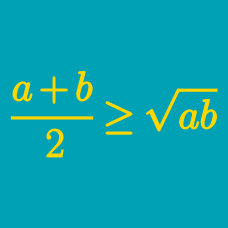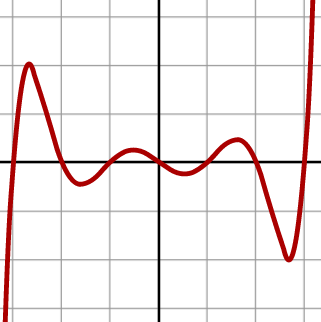Algebra

# Power Mean Inequalities: Level 5 Challenges

$\large P=(x+y)(z+t)$

Let $x,y,z,t$ be positive real numbers satisfying $x^2+y^2+z^2+t^2=10$ and $xyzt=4$.

If the maximum value of the expression is $S$, find $\lfloor 100 S \rfloor$.

$\sum_{k=1}^{n} x_k^2 \le \sum_{k=1}^n \dfrac{1}{x_k^2}$

Given that $x_1,x_2, \ldots,x_n$ are positive reals whose sum is $n$, find the largest integer $n$ such that the inequality above always holds true.

If you think all positive integers $n$ make the inequality hold true, enter 0 as your answer.

$\large \frac{3(b+c)}{2a} + \frac{4a+3c}{3b} + \frac{12(b-c)}{2a+3c}$

If $a,b$ and $c$ are positive real numbers, find the minimum value of the expression above.

Bonus: Find the values of $a,b$ and $c$ when the expression above is minimized.What is the minimum value of $p(2)$ if the following 4 conditions are followed?

1. $p(x)$ is a polynomial of degree $17$.

2. All roots of $p(x)$ are real.

3. All coefficients are positive.

4. The coefficient of $x^{17}$ is 1.

5. The product of roots of $p(x)$ is -1.

##### Image Credit: Wikimedia Septic Graph

$\large 16x^2 +y^2 + \frac 1{x^2} + \frac y{2x}$

Non-zero real numbers $x$ and $y$ are such that the minimum value of expression above can be expressed as $\sqrt m$, then what is $m$?

×